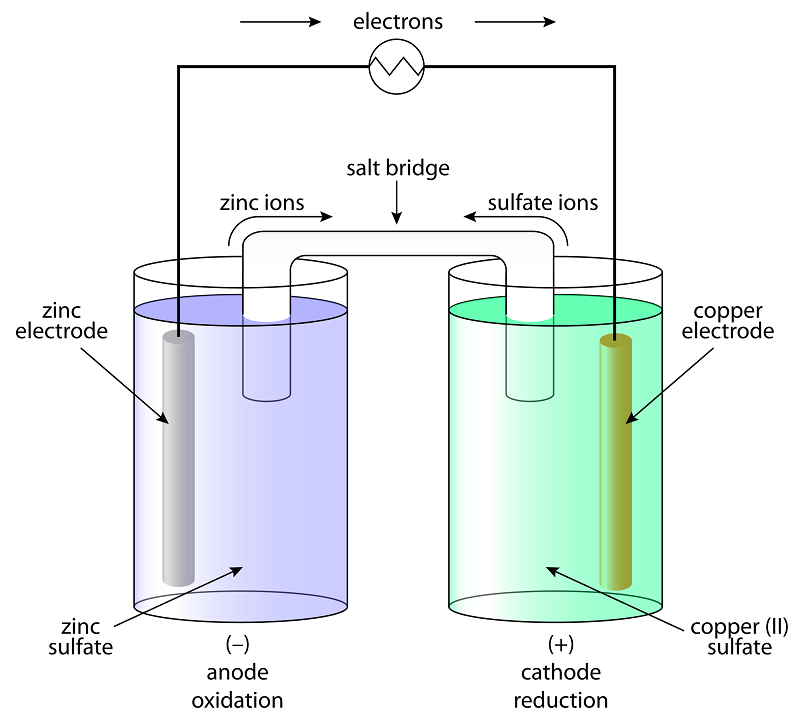# Gibbs Free Energy - Relation Emf of a cell and Gibbs free energy

## What is Gibbs free energy?

Gibbs Free Energy is defined as the thermodynamic potential that signifies the maximum or reversible work performed by a thermodynamic system at constant temperature and pressure. Work done by electrical power in one second is given as the product of emf of the cell and the total charge passed.

## Gibbs free energy equation

ΔG = ΔH – TΔS

where,

ΔG → Gibbs free energy

ΔH → enthalpy change

T → temperature

ΔS → change in entropy.

 Gibbs free energy Reaction ΔG < 0 Spontaneous ΔG > 0 Non-spontaneous ΔG = 0 Equilibrium

## Gibbs free energy and emf of the cell

W = nFE (cell)

Where,

w → work done,

nF → total charge passed and

E(cell) emf of the cell

When the charge is passed reversibly through the galvanic cell, it is observed that maximum work is done by the galvanic cell. This reversible work done by the galvanic cell is related to the decrease in Gibbs energy of the reaction.Gibbs free energy – Experiment

Δr G = W

Δr G = nFE(cell)

The above equation can also be used to calculate the standard cell potential. When the concentration of all the reacting species is unity then E(cell) = Eo(cell) (emf of a cell is equal to standard cell potential).

Δr Go = nFEo(cell)

Gibbs energy of a reaction is an Extensive Thermodynamic Property which means its value depends on n. Thus, for two cell reactions involving different values of n, we observe different values of Gibbs free energy of the reaction. For example:

Zn(s) + Cu2+(aq) → Zn2+(aq) + Cu(s)

Δr G = 2FE(cell)

Whereas after multiplying RHS and LHS by 2 we notice,

2Zn(s) + 2Cu2+(aq) → 2Zn2+(aq) +2Cu(s)

Δr G = 4FE(cell)

According to thermodynamics, Gibbs energy of a reaction can be related to the reaction quotient and when the reaction is at equilibrium it can be related to the equilibrium constant. Since Gibbs energy of a reaction also depends on the emf of a cell we can relate the equilibrium constant of a cell reaction with the standard cell potential. At equilibrium,

Δr Go = nFEo(cell)

ΔrGo =   RT ln K

nFEo(cell)  RT ln K

Eo(cell) = (RT/nF) ln K

Where,

K → equilibrium constant

R → universal gas constant

From the above equation, we can infer:

For K > 1, Eo(cell)> 0, the reaction is spontaneous and favours the formation of the product.

For K < 1, Eo(cell<0, the reaction is non-spontaneous and favours the formation of reactant.

### Solved example

1. Calculate the standard free energy change and standard emf of the cell reaction for Zn, Zn2+ || Ni2+, Ni.

The standard electrode potential of Zn2+, Zn and Ni2+

The standard reduction potentials of Zn2+, Zn and Ni2+, Ni half cells are as follows -0.76 V and -0.25 V.

Ecell  =   ER – EL = – 0.25 – (- 0.76)

= + 0.51 V  Eocell is + ve. \ DGo = – ve.

DG=  – n F Ecell

n = 2 electrons

DGo = (-2) (96495) (0.51)

= – 98425 J = – 98.425

## What is meant by Gibbs free energy?

The energy of a chemical reaction which can be used to do work.

## What is Gibbs free energy used for?

To measure the potential for reversible and maximum work that can be done by the system at constant pressure and temperature.

## How is Gibbs free energy mathematically expressed?

G = H – TS

where,

H → enthalpy

T → temperature and

S → entropy.

Learn about Gibbs Energy Change: Spontaneity of a Process with BYJU’S -The Learning App.